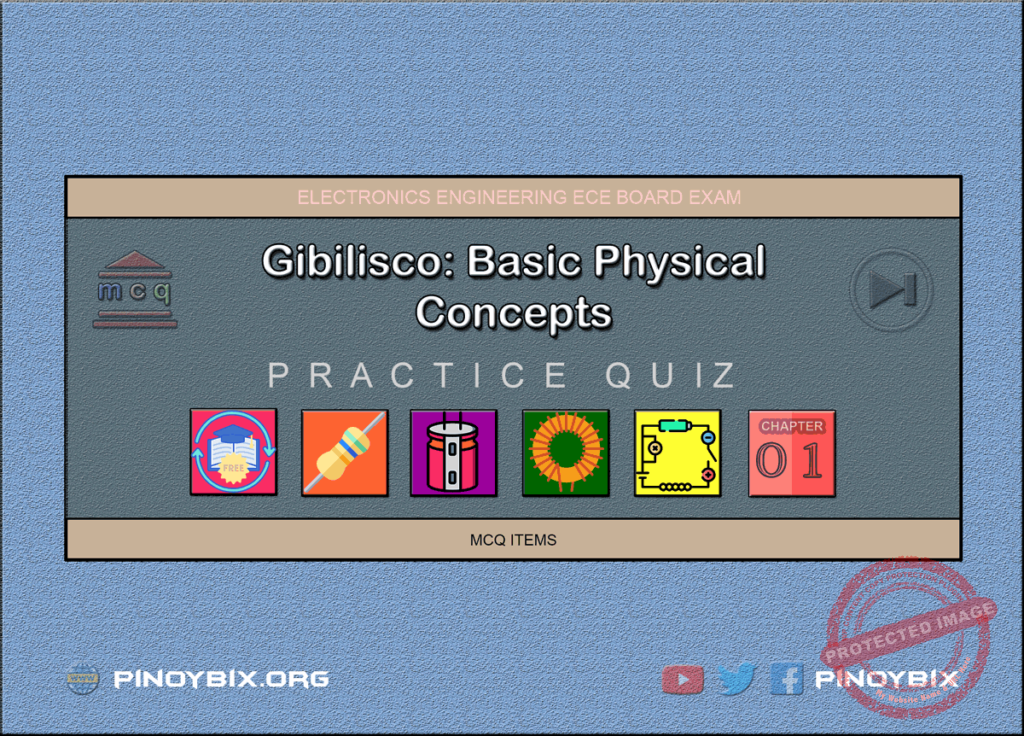# Gibilisco: MCQ in Basic Physical Concept | ECE Board Exam

(Last Updated On: April 2, 2022)This is the Multiple Choice Questions (MCQs) in Chapter 1: Basic Physical Concept from the book Teach Yourself Electricity and Electronics, 5th edition by Stan Gibilisco. If you are looking for a reviewer in Electronics Engineering this will definitely help you before taking the Board Exam.

Begin the Test

Choose the letter of the best answer in each questions.

1. The atomic number of an element is determined by:

A. The number of neutrons.

B. The number of protons.

C. The number of neutrons plus the number of protons.

D. The number of electrons.

Solution:

2. The atomic weight of an element is approximately determined by:

A. The number of neutrons.

B. The number of protons.

C. The number of neutrons plus the number of protons.

D. The number of electrons.

Solution:

3. Suppose there is an atom of oxygen, containing eight protons and eight neutrons in the nucleus, and two neutrons are added to the nucleus. The resulting atomic weight is about:

A. 8.

B. 10.

C. 16.

D. 18.

Solution:

4. An ion:

A. Is electrically neutral.

B. Has positive electric charge.

C. Has negative electric charge.

D. Might have either a positive or negative charge.

Solution:

5. An isotope:

A. Is electrically neutral.

B. Has positive electric charge.

C. Has negative electric charge.

D. Might have either a positive or negative charge.

Solution:

6. A molecule:

A. Might consist of just a single atom of an element.

B. Must always contain two or more elements.

C. Always has two or more atoms.

D. Is always electrically charged.

Solution:

7. In a compound:

A. There can be just a single atom of an element.

B. There must always be two or more elements.

C. The atoms are mixed in with each other but not joined.

D. There is always a shortage of electrons.

Solution:

8. An electrical insulator can be made a conductor:

A. By heating.

B. By cooling.

C. By ionizing.

D. By oxidizing.

Solution:

9. Of the following substances, the worst conductor is:

A. Air.

B. Copper.

C. Iron.

D. Salt water.

Solution:

10. Of the following substances, the best conductor is:

A. Air.

B. Copper.

C. Iron.

D. Salt water.

Solution:

11. Movement of holes in a semiconductor:

A. Is like a flow of electrons in the same direction.

B. Is possible only if the current is high enough.

C. Results in a certain amount of electric current.

D. Causes the material to stop conducting.

Solution:

12. If a material has low resistance:

A. It is a good conductor.

B. It is a poor conductor.

C. The current flows mainly in the form of holes.

D. Current can flow only in one direction.

Solution:

13. A coulomb:

A. Represents a current of one ampere.

B. Flows through a 100-watt light bulb.

C. Is one ampere per second.

D. Is an extremely large number of charge carriers.

Solution:

14. A stroke of lightning:

A. Is caused by a movement of holes in an insulator.

B. Has a very low current.

C. Is a discharge of static electricity.

D. Builds up between clouds.

Solution:

15. The volt is the standard unit of:

A. Current.

B. Charge.

C. Electromotive force.

D. Resistance.

Solution:

16. If an EMF of one volt is placed across a resistance of two ohms, then the current is:

A. Half an ampere.

B. One ampere.

C. Two amperes.

D. One ohm.

Solution:

17. A backwards-working electric motor is best described as:

A. An inefficient, energy-wasting device.

B. A motor with the voltage connected the wrong way.

C. An electric generator.

D. A magnetic-field generator.

Solution:

18. In some batteries, chemical energy can be replenished by:

A. Connecting it to a light bulb.

B. Charging it.

C. Discharging it.

D. No means known; when a battery is dead, you have to throw it away.

Solution:

19. A changing magnetic field:

A. Produces an electric current in an insulator.

B. Magnetizes the earth.

C. Produces a fluctuating electric field.

D. Results from a steady electric current.

Solution:

20. Light is converted into electricity:

A. In a dry cell.

B. In a wet cell.

C. In an incandescent bulb.

D. In a photovoltaic cell.

Solution:

### Topics Included in the Test from Chapter 1 to Chapter 8

Part 1: Direct Current
Chapter 6: MCQ in Resistors
Chapter 8: MCQ in Magnetism

#### Complete List of Chapter Quiz in Teach Yourself Electricity and Electronics

PinoyBIX Engineering. © 2014-2021 All Rights Reserved | How to Donate? |